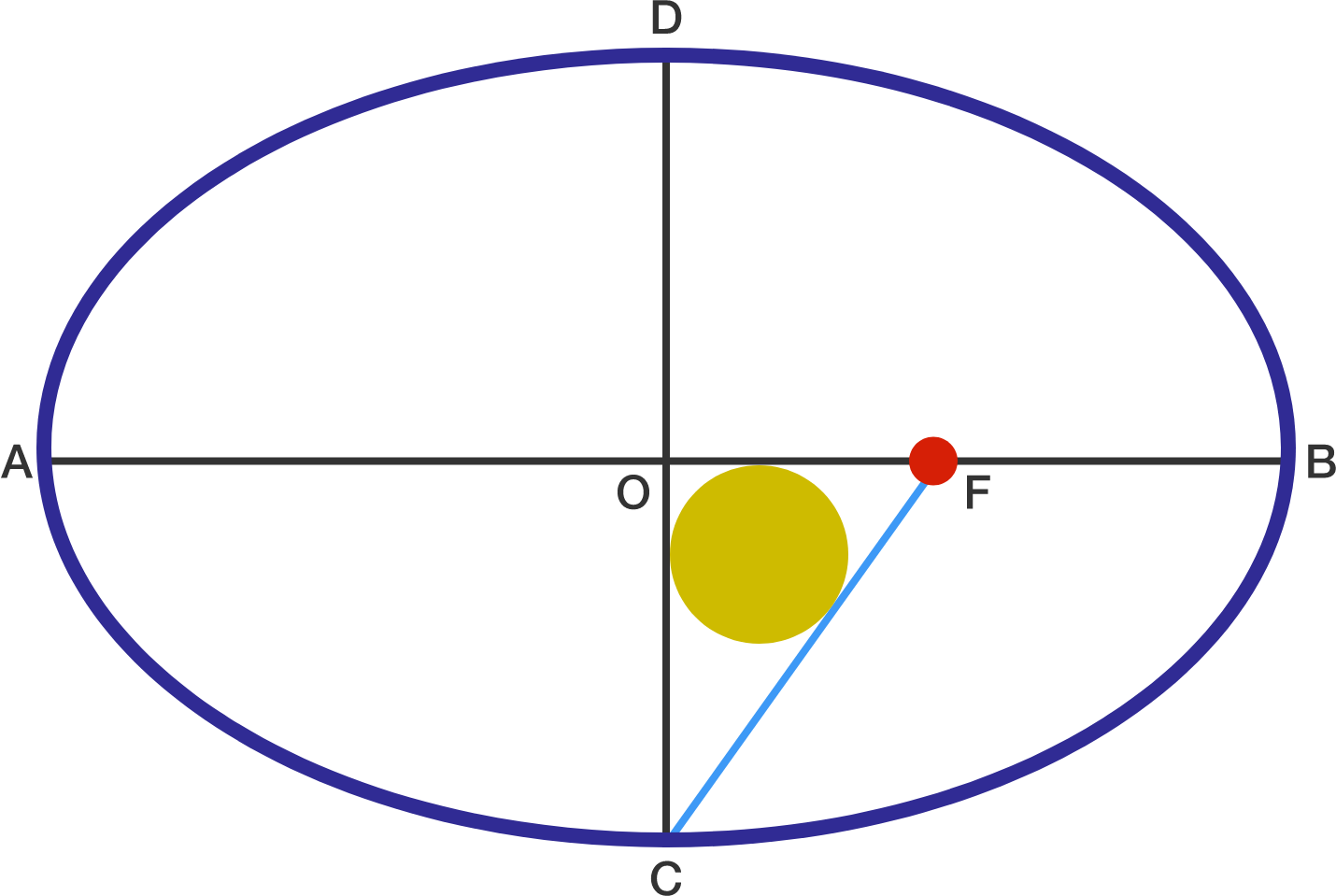# Cool Ellipse

Geometry Level 2Point $O$ is the center of the ellipse with major axis $AB$ & minor axis $CD$ Point F is one of the focus of this ellipse.

If $OF=6$, and the diameter of inscribed circle of triangle $\triangle{OCF}$ is $2$, then find $(AB)\cdot (CD)$

×